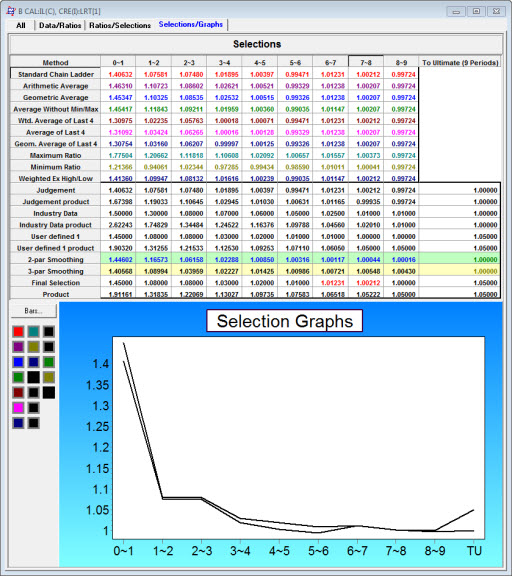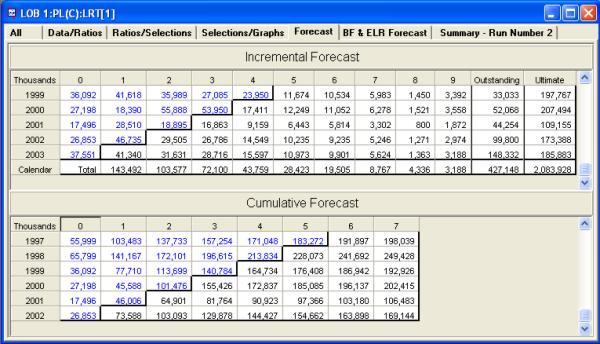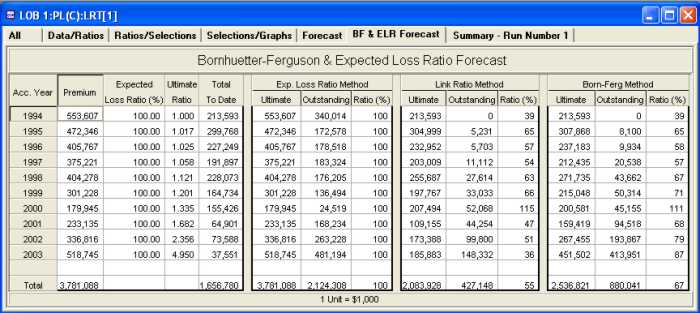Both ICRFS™ and ELRF™ contain a Link Ratio Techniques (LRT) module. In this module, standard actuarial methods can be applied to the data. Link ratios can be selected easily, and alternative link ratio methods can be compared either in table format or graphically. A further example of link ratio methods is available here.

The standard link ratio methods as calculated automatically in the LRT module are:

• Chain Ladder (Volume Weighted Average)
• Arithmetic Average
• Geometric Average
• Average Without Min/Max
• Last N Diagonal Weighted Average
• Last N Diagonal Average
• Last N Diagonal Geometric Average
• Maximum Ratio
• Minimum Ratio
• Two parameter smoothing
• Three parameter smoothing

By default N is set to be 4 periods, but N can be changed to any calculable value. A ratio 'to ultimate' can also be set.

Furthermore, link ratios can be edited directly for the final selection.

An example screen shot from the module is shown below:Different link ratio methods can be used in different development periods if you choose. The number of calendar periods included in the average can also be set.

Forecasting with link ratios produces a table like the following:### Bornhuetter-Ferguson results available instantly

When you forecast with link ratios, the Expected Loss Ratio and Bornhuetter-Ferguson forecasts are also calculated - as illustrated in the table below.A further example of link ratio methods is available here.

(c) Insureware 1985 ~ 2023

Insureware Pty. Ltd.
info@insureware.com